Data Consolidation through Excel VBA

In this article, we are going to learn VBA how to write the macro to consolidate the data in Microsoft Excel.

Consolidate Data: -This option is used to summarize data from separate ranges, consolidating the results in single output.

Let’s take an example to understand how to combine the data through VBA.

We have 3 Excel Workbooks, named as A, B, and C. Every workbook has Sheet1 with the numbers in the range A2:A4. Now, we want to retrieve the sum to all workbooks in an Excel file with the sum of numbers and file name as well.

File A:-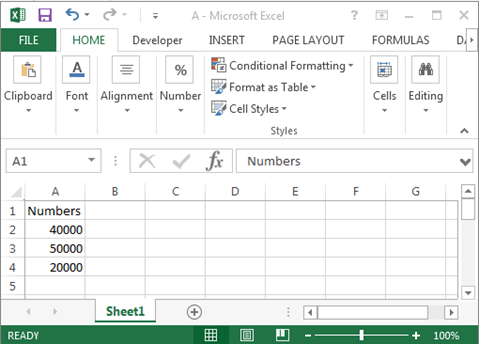File B:-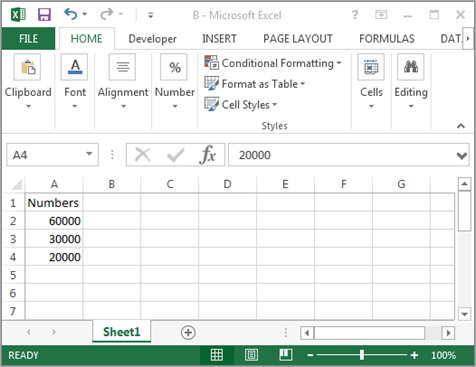File C:-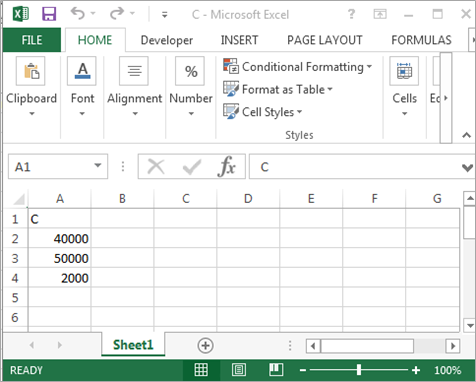Consolidate:-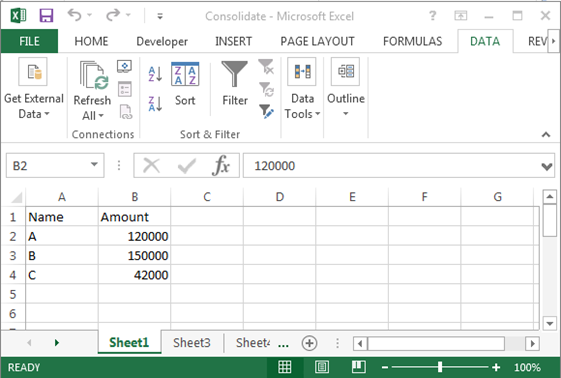To consolidate the data, follow below given steps:-

• Insert a User Form, then insert 3 label boxes, 3 text boxes and one command button.
• Double click on Command button to assign the Macro.
• Write the below mentioned code:-

```Sub Consolidate()
Range("A1").Select
ActiveCell.Value = "Name"
Range("B1").Select
ActiveCell.Value = "Amount"
Range("A2").Select
ActiveCell.Value = "A"
Range("A3").Select
ActiveCell.Value = "B"
Range("A4").Select
ActiveCell.Value = "C"Range("B2").SelectWorkbooks.OpenFileName:="D:\Data\A.xlsx"
Workbooks.OpenFileName:="D:\Data\B.xlsx"
Workbooks.OpenFileName:="D:\Data\C.xlsx"Windows("Consolidate").Activate
Selection.Consolidate Sources:=Array("'D:\Data\[A.xlsx]sheet1'!R2C1:R4C1", _
"'D:\Data\[B.xlsx]sheet1'!R2C1:R4C1", "'D:\Data\[C.xlsx]sheet1'!R2C1:R4C1"), Function:=xlSum
Windows("A.xlsx").Activate
ActiveWorkbook.Close
Windows("B.xlsx").Activate
ActiveWorkbook.Close
Windows("C.xlsx").Activate
ActiveWorkbook.Close
End Sub
```

Code Explanation:-Firstly, we will define the range values and then we will define the path of every file. Then we will define that which function will work for every file and what calculation we want to consolidate.
• To run the code, press F5 key.
• All files will be opened and then VBA will return the Sum to all files and then will put the result in Front of file name.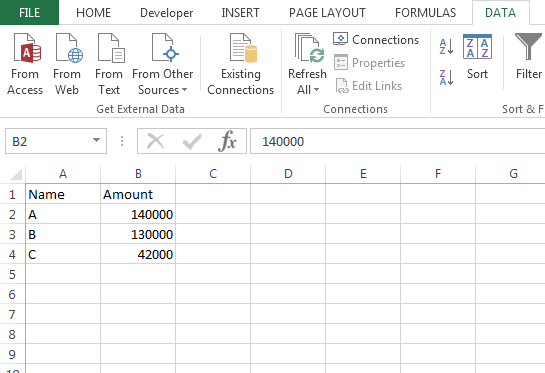This is the way we can consolidate the numbers and retrieve in single file from different files in Microsoft Excel through VBA.